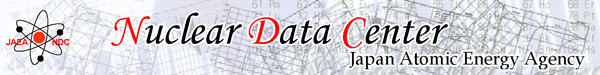Usage of VARCS

Average capture cross sections are plotted on a graph. The data are based on JENDL-4.0 and are broadened with 293.6 K by using LINEAR, RECENT and SIGMA1 codes. If an updated data exists in JENDL-4.0u, the data will be applied to the graph.

~ How to operate ~
[Target] input
A target nuclide name. (e.g., Am-241, Am-242m)
[Reaction] list
A list of neutron-induced reaction. The default reaction is neutron capture (MT=102).
[Flight Length] input
Neutron flight length in meter unit for measurement by time-of-flight (TOF) method. Inputting 0 [nsec/ch] (or a negative value) is not available. The default value is 21.5 [m]. This input is ineffective when “Energy [eV]” is selected in the [Graph Unit & Range] input.
[Channel Width] input
Time per channel in nanosecond unit. Inputting 0 [nsec/ch] (or a negative value) is not available. The default value is 200 [nsec/ch]. This input is ineffective when “Energy [eV]” is selected in the [Graph Unit & Range] input.
[T = Tm - T0] input
A value of time offset, T0 , in the above equation can be input in nanosecond unit. The default value is 0 [nsec]. This input is ineffective when “Energy [eV]” is selected in the [Graph Unit & Range] input.
[Grids to Calculate the Average] input
Grid points of X-axis to average cross sections can be input by channel number or energy in [eV] unit according to the [Graph Unit & Range] input. Input values should be separated by blanks, commas or carriage returns. Incremental input is also available to use sets of 3 values, start point, end point and increment, enclosed in parenthesis such as (START END INCR). Default grids are also available by clicking the [Set Default] button. All input values are deleted by clicking the [Clear] button.
[(Re-) Draw Graph] button
A (new) graph will be (re-)drawn by clicking this button corresponding to input condition. The graph image is a PNG formatted file, and is 480×360 pixel size on the operating window. However, the actual image is 640×480 pixel size, and will be shown on another window or tab by clicking the image on the operating window.
[Average Numerical Data]
Tabulated numerical data used to plot the graph are shown on another window or tab by clicking the "Average Numerical Data" link just below the graph. The table is constructed with the lower and upper boundaries of averaged range in channel number or energy [eV], and average cross sections [barn].
[Graph Unit & Range] input
Unit of X-axis can be selected from channel unit (Channel) and incident energy unit (Energy [eV]). The default is channel unit.
Range of graph can be set by inputting range of X-axis and Y-axis. If nothing is input, the range will be automatically set.